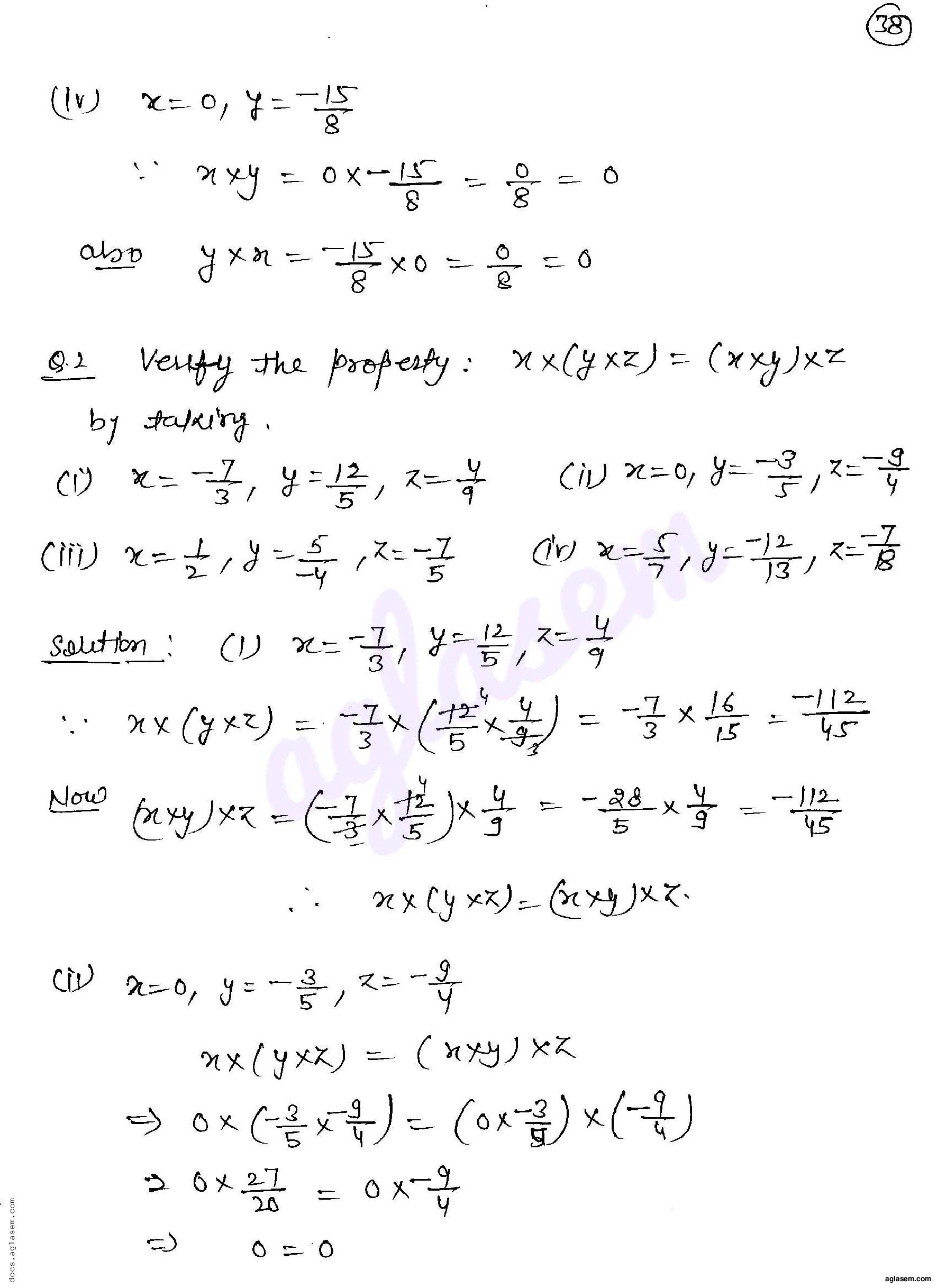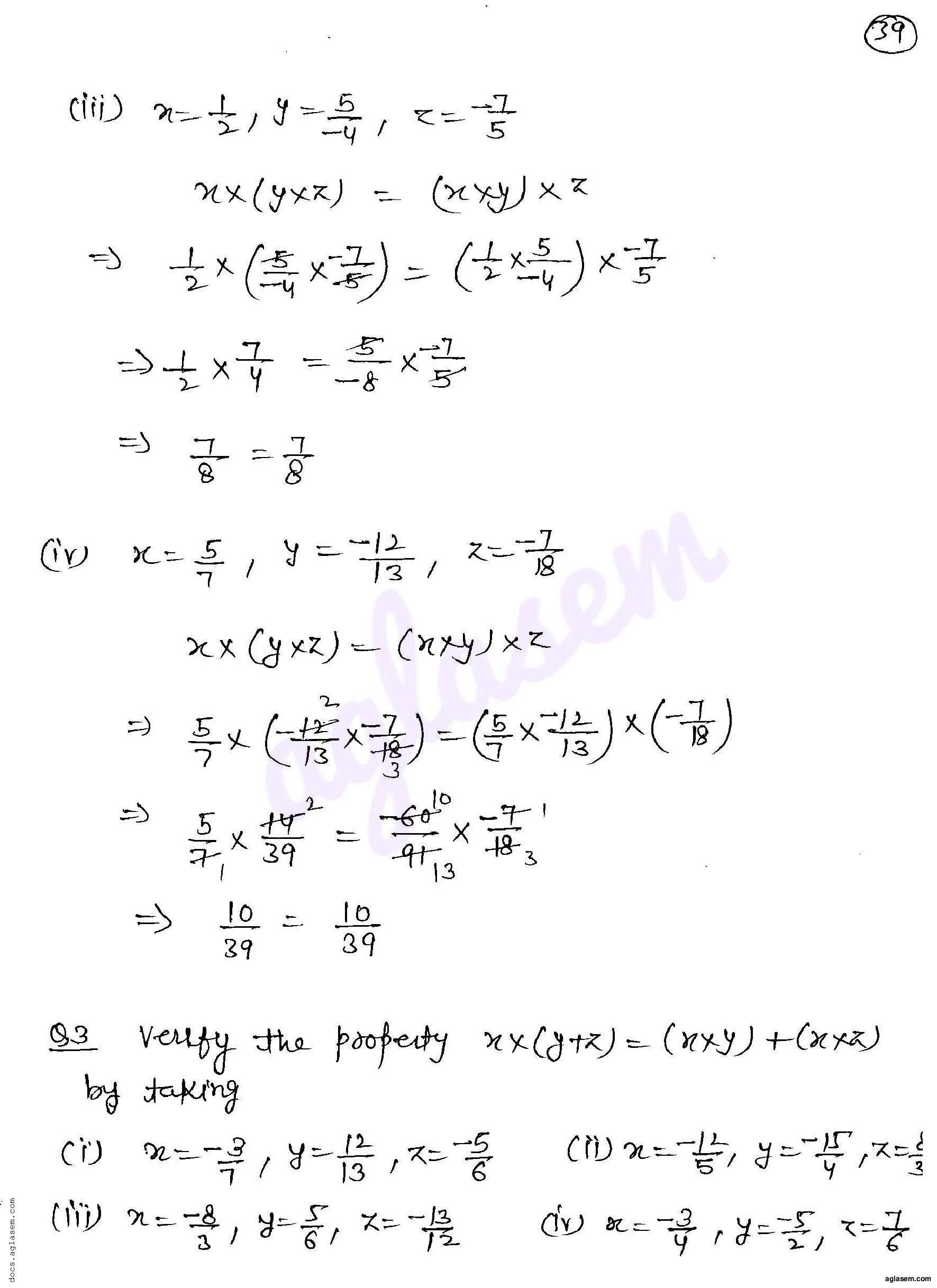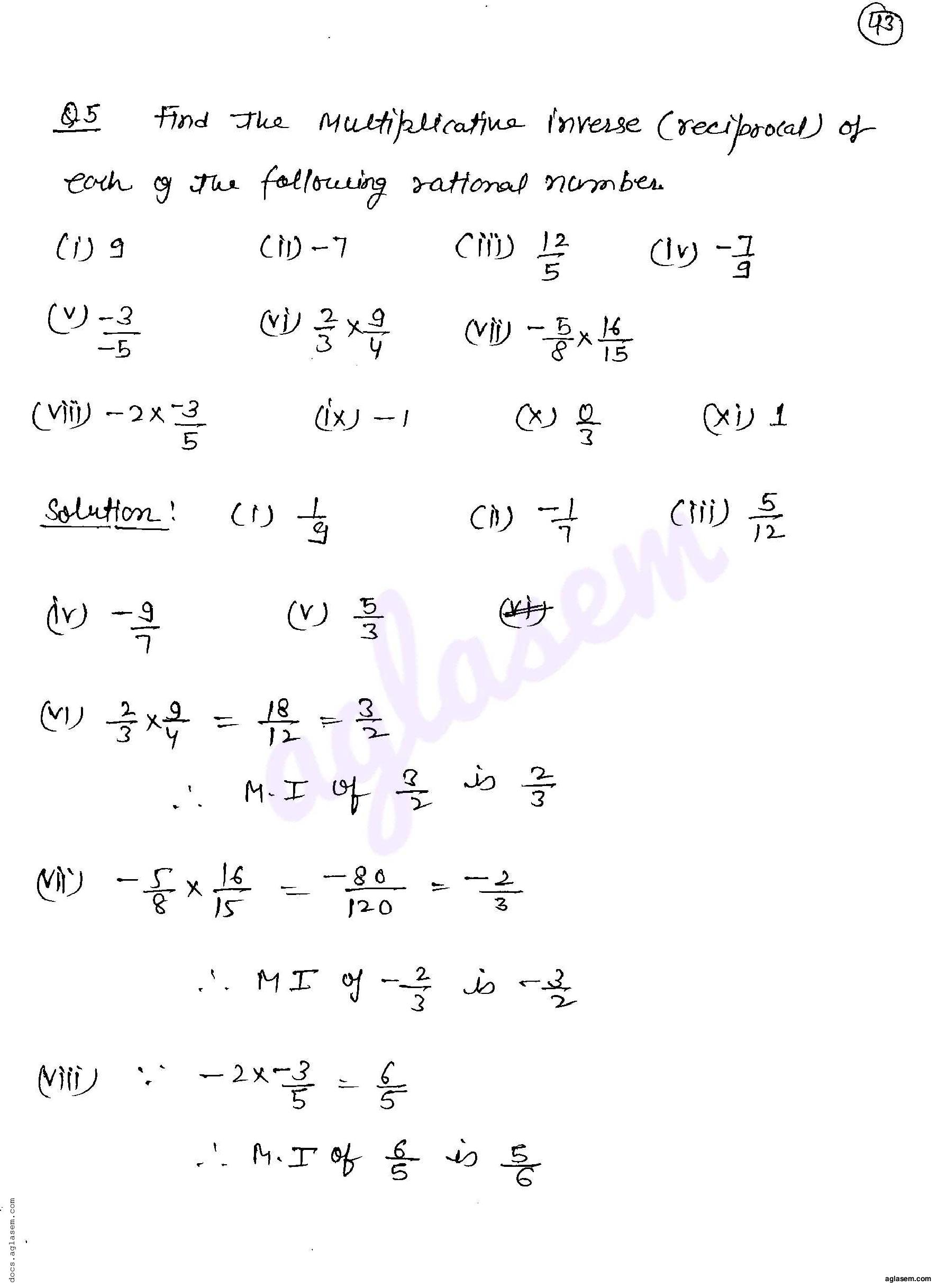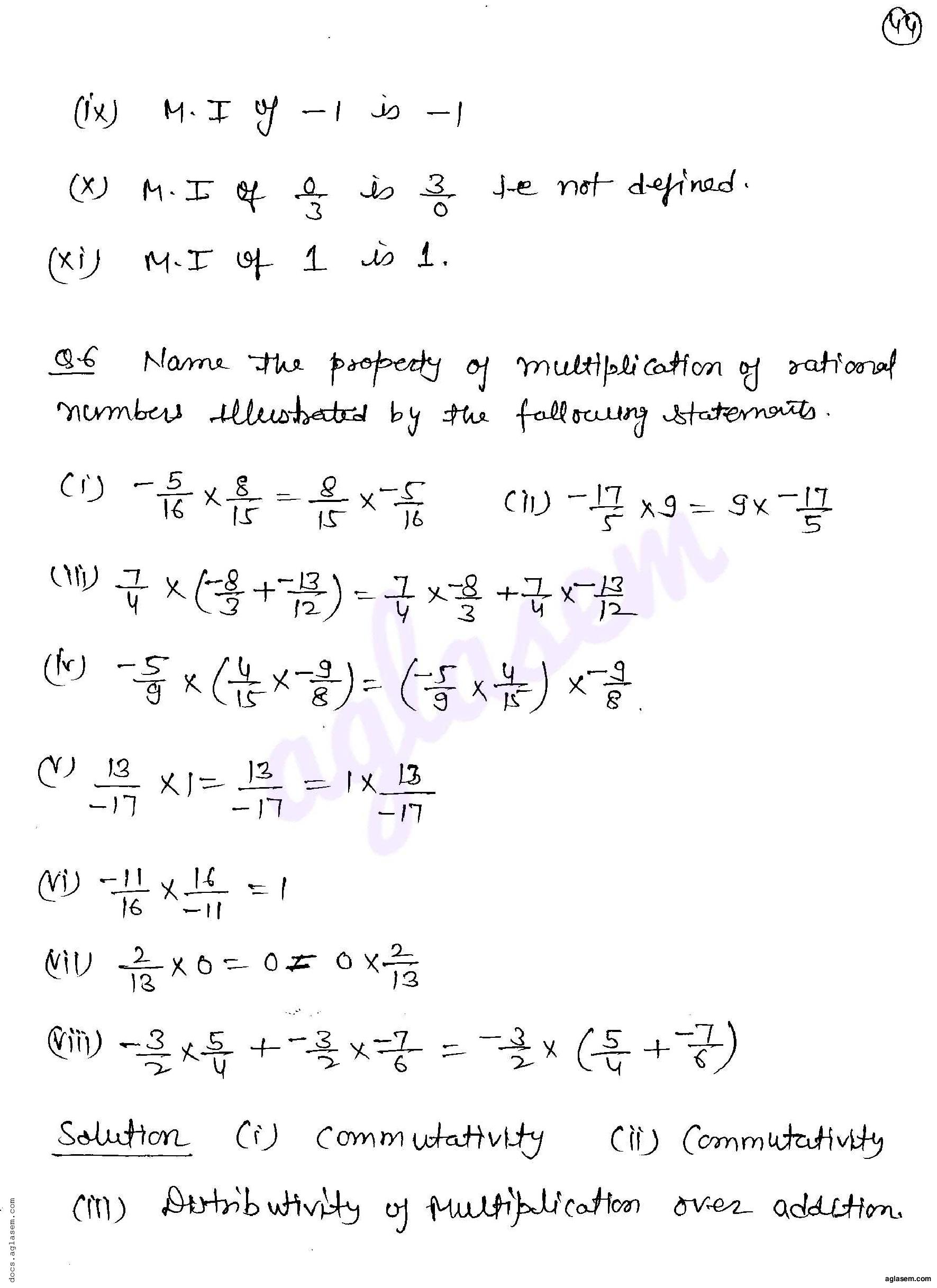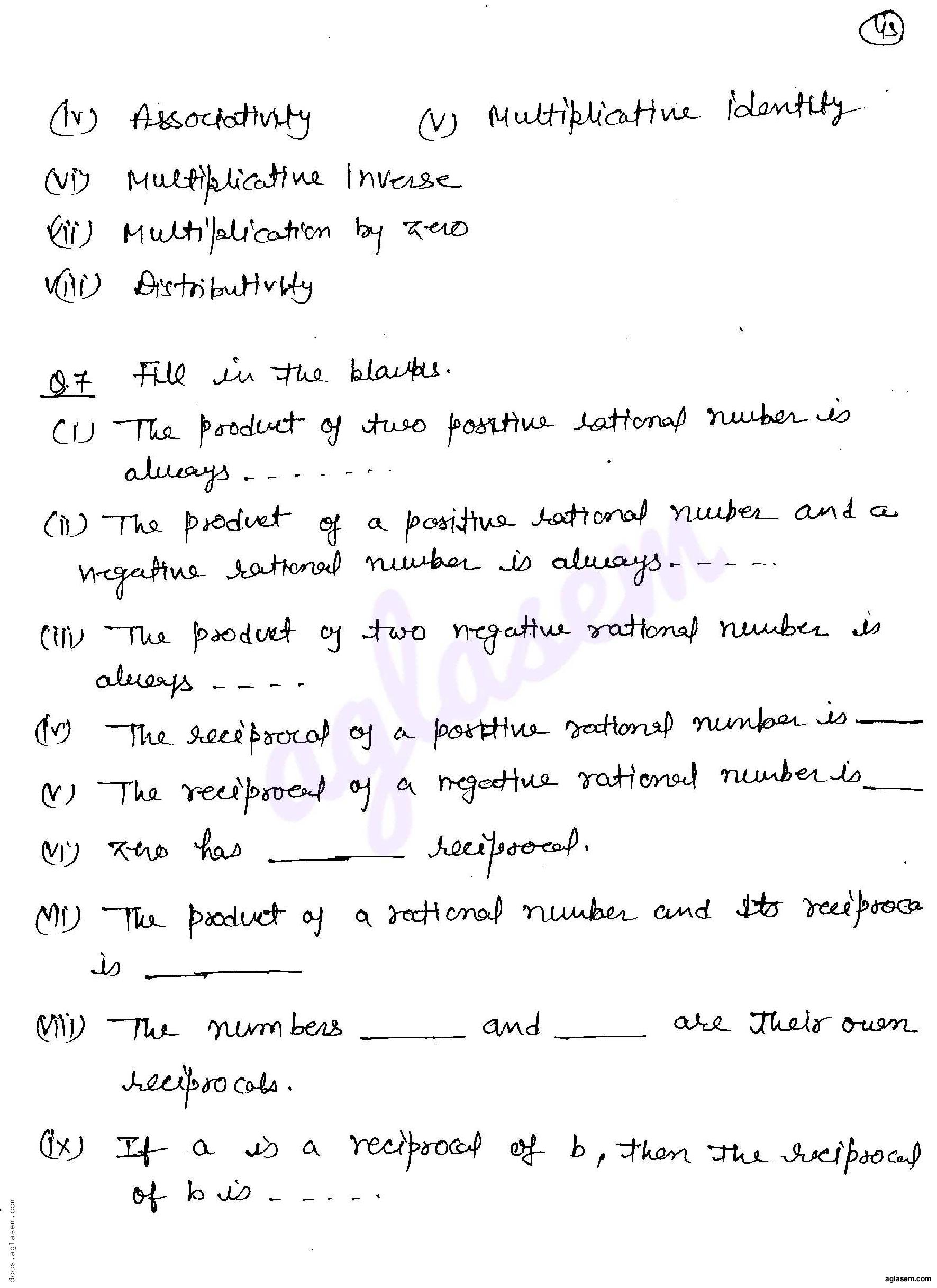# RD Sharma Solutions Class 8 Chapter 1 Rational Numbers Exercise 1.6

Here you can get free RD Sharma Solutions for Class 8 Maths Chapter 1 Rational Numbers Exercise 1.6. All RD Sharma Book Solutions are given here exercise wise for the chapter Rational Numbers. RD Sharma Solutions are helpful in the preparation of several school level, graduate and undergraduate level competitive exams. Practicing questions from RD Sharma Mathematics Solutions for Class 8 Chapter 1 Rational Numbers is proven to enhance your math skills.

 Class: Class 8th Chapter: Chapter 1 Exercise: Exercise 1.6 Name: Rational Numbers

## RD Sharma Solutions Class 8 Chapter 1 Rational Numbers Exercise 1.6

RD Sharma Class 8 Solutions Chapter 1 for Rational Numbers Exercise 1.6 are given below.

RD Sharma Solutions Class 8 Chapter 1 Rational Numbers Exercise 1.6